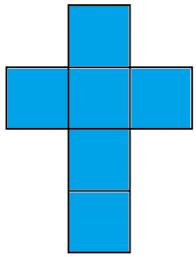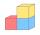# Cube wall

The perimeter of one cube wall is 120 meters. Calculate the surface area and the body diagonal of this cube.

S =  5400 m2
u =  51.9615 m

### Step-by-step explanation:Did you find an error or inaccuracy? Feel free to write us. Thank you!Tips to related online calculators
Pythagorean theorem is the base for the right triangle calculator.

#### You need to know the following knowledge to solve this word math problem:

We encourage you to watch this tutorial video on this math problem:

## Related math problems and questions:

• Cube wallCalculate the cube's diagonal if you know that one wall's surface is equal to 36 centimeters square. Please also calculate its volume.
• Cube diagonalsThe cube has a wall area of 81 cm square. Calculate the length of its edge, wall, and body diagonal.
• Wall diagonalCalculate the length of wall diagonal of the cube whose surface is 384 cm square.
• Body diagonal - cubeCalculate the surface and cube volume with body diagonal 15 cm long.
• Cube diagonalsDetermine the volume and surface area of the cube if you know the length of the body diagonal u = 216 cm.
• Body diagonalCalculate the volume and surface of the cube if the body diagonal measures 10 dm.
• Body diagonalCalculate the cube volume, whose body diagonal size is 75 dm. Draw a picture and highlight the body diagonal.
• Cube and sphereCube with the surface area 150 cm2 is described sphere. What is sphere surface?
• Surface and volume - cubeFind the surface and volume of a cube whose wall diagonal is 5 cm long.
• Body diagonalCalculate the length of the body diagonal of the 6cm cube.
• Regular square prismThe volume of a regular square prism is 192 cm3. The size of its base edge and the body height is 1: 3. Calculate the surface of the prism.
• Cuboid diagonalsThe cuboid has dimensions of 15, 20 and 40 cm. Calculate its volume and surface, the length of the body diagonal and the lengths of all three wall diagonals.
• Body diagonalFind the cube surface if its body diagonal has a size of 6 cm.
• Space diagonal anglesCalculate the angle between the body diagonal and the side edge c of the block with dimensions: a = 28cm, b = 45cm and c = 73cm. Then, find the angle between the body diagonal and the plane of the base ABCD.
• CalculateCalculate the length of the wall diagonal of the cube with an edge 5 cm long.
• Cube diagonalsCalculate the length of the side and the diagonals of the cube with a volume of 27 cm3.
• Four sided prismCalculate the volume and surface area of a regular quadrangular prism whose height is 28.6cm and the body diagonal forms a 50-degree angle with the base plane.